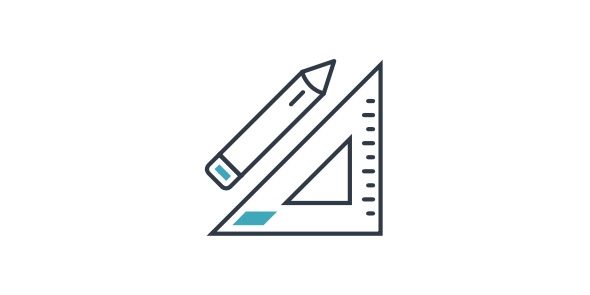# Online Quiz On 3rd Grade Math

15 Questions | Total Attempts: 144SettingsHow much do you really know about US states? Find out by taking this quiz!

• 1.
What is 8000 + 70 + 6?
• A.

8706

• B.

8760

• C.

8067

• D.

8076

• 2.
What is 3 thousands + 6 hundreds + 7 tens + 3 ones?
• A.

3763

• B.

3637

• C.

3673

• D.

3736

• 3.
In the problem 8 divided by 4 = 2, what do we call the 2?
• A.

Dividend

• B.

Divisor

• C.

Quotient

• D.

Product

• 4.
Which statement is not true?
• A.

490 > 904

• B.

68 < 71

• C.

3 < 6

• D.

428 > 417

• 5.
What number must be multiplied by 5 to get 20?
• A.

4

• B.

20

• C.

5

• D.

1

• 6.
If your store has 376 boxes of candy on Monday and 52 on Friday, how many were sold?
• A.

52

• B.

324

• C.

314

• D.

376

• 7.
If Jake has \$19, how much change will he have left after he buys a pair of gloves for \$17.95?
• A.

2.05

• B.

1.05

• C.

0.05

• D.

0.95

• 8.
How do you write 80 + 300 in standard form?
• A.

830

• B.

380

• C.

803

• D.

308

• 9.
What is 15 + 18?
• A.

53

• B.

33

• C.

43

• D.

23

• 10.
What is 24 + 4 + 22 + 4?
• A.

54

• B.

64

• C.

50

• D.

46

• 11.
What is 78 - 36?
• A.

42

• B.

32

• C.

52

• D.

62

• 12.
What number must be multiplied by 5 to get 35?
• A.

6

• B.

5

• C.

8

• D.

7

• 13.
What is the remainder when 129 is divided by 30?
• A.

30

• B.

3

• C.

9

• D.

4

• 14.
How do you write 0.46 as a fraction?
• A.

46/100

• B.

46/1

• C.

0.46/100

• D.

4.6/100

• 15.
What number must be multiplied by 7 to get 21?
• A.

21

• B.

3

• C.

3-Jan

• D.

7

Related Topics